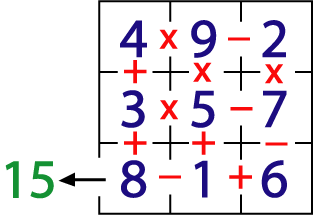#### You may also like### Exploring Wild & Wonderful Number Patterns

EWWNP means Exploring Wild and Wonderful Number Patterns Created by Yourself! Investigate what happens if we create number patterns using some simple rules.### Sending Cards

This challenge asks you to investigate the total number of cards that would be sent if four children send one to all three others. How many would be sent if there were five children? Six?### Dice and Spinner Numbers

If you had any number of ordinary dice, what are the possible ways of making their totals 6? What would the product of the dice be each time?

# A-magical Number Maze

##### Age 7 to 11Challenge Level

The rows, columns and main diagonals of a $1$ - $9$ magic square add to the same number, $15$.

This magic square has had doors, with operations written in them, opened to make it into a maze.Start wherever you like and go through every cell using all the numbers, doing the operation indicated to the numbers, and go out through the exit marked in cell $8$ with a total of $15$!

You must use the operations and numbers strictly in the order you meet them. For example, if you try starting at $4$ and go across the top row you must do it like this:

$4\times 9 = 36$

$36 - 2 = 34$

NOT like this:

$4 \times (9 -2) = 4 \times 7 =28$